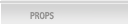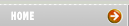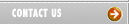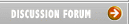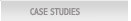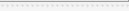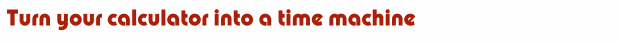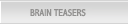Author:  Lori Alden Audience:  Advanced placement and college economics students Time required:  About 30 minutes NCEE Standards:  12, 15 Summary:  This activity teaches students how to do continuous compounding using the ex button on their scientific calculators.  The problems at the end of the activity vividly demonstrate how small changes in a country's growth rate can drastically affect the standard of living of future generations. Reading: Nestled among the rows of buttons on any scientific calculator is one labeled ex. Punching it takes any value x that's displayed in your calculator's window and raises e (a number equal to about 2.7) to that power.  The button's purpose?  It lets numbers travel through time. Here's how it works.  Suppose you're putting \$1,000 into a savings account that pays 7% interest per year.  How much will your account be worth in 5 years? The formula we'll use is this: Value in D = Value in S × er × (D ─ S) S represents the starting year of the number's voyage through time (2005), D is the destination year (2010), and r represents the percentage rate at which your savings account will grow (7% or .07).  Your account is now worth \$1,000--this is its Value in S.  We want to find your account's Value in D. Start by finding the value of e's exponent in the equation. r × (D - S) = .07 × (2010 - 2005) = .35 Now punch the ex key. e.35 = 1.419 Next, multiply 1.419 by the account's Value in S, \$1,000. \$1,000 × 1.419 = \$1,419 Your account will grow to \$1,419 in 5 years. You can go back in time with the ex key, too.  Suppose that your family's house has been growing in value by 4% per year over the past 20 years.  It's worth \$100,000 today.  How much was it worth 20 years ago? In this problem, the starting year is 2005 and the destination year is 1985.  Again, start by calculating e's exponent: r × (D - S) = .04 × (1985 - 2005) = -.8 Next, punch the ex key and multiply the result by the Value in S, \$100,000. Value in D = \$100,000 × e─.8 = \$44,933 Your house was worth \$44,933 in 1985. The ex key works with any value that grows (or shrinks) at a constant percentage rate r.  This means it can be used to calculate how population, GNP, prices, and many other values change over time.            Problems 1. Real GWP (gross world product) in 1 A.D. is estimated to have been roughly \$18.5 billion (in 1990 dollars).   Had the economies of the world experienced a steady growth rate of 1% per year since then, what would real GWP be today?  If the world's population is roughly 6.5 billion, what would real per capital GWP be today? Solution:  Value in 2005 = Value in 1 AD × e.01 × (2005 ─ 1) Value in 2005 = \$18.5 billion × 504,965,162.6 Value in 2005 = \$9,341,855,509 billion Per capita value in 2005 = \$9,341,855,509 billion/6.5 billion = \$1,437,208,540 = \$1.437 billion per person on the planet. (This amazing result illustrates the power of compounding--had mankind not experienced the low-growth dark ages, all of us would have been much richer.) 2.  In 1999, the U.S. per capita GDP was about \$32,500.  If we experience 2% real annual growth from 1999 to 2024, what will our per capital GDP be in 2024?  Solution:  Value in 2024 = Value in 1999 × e.02 × (2024 ─ 1999)  Value in 2024 = \$32,500 (1.6487) = \$53,583   3.  Two countries start with equal GDPs.  But Country A grows at an annual rate of 2 percent while Country B grows at an annual rate of 2.5 percent.  After 25 years, how much larger than Country A is Country B? Country A:  Value in 2030 = Value in 2005 × e.02 × (2030 ─ 2005) = Value in 2005 (1.6487) Country B:  Value in 2030 = Value in 2005 × e.025 × (2030 ─ 2005) = Value in 2005 (1.8682) Country B in 2030/Country A in 2030 = Value in 2005 (1.8682)/Value in 2005 (1.6487) = 1.133 Country B is 13.3% larger.     © Lori Alden, 2005-7.  All rights reserved.  You may download the content, provided you only use the content for your own personal, non-commercial use.  Lori Alden reserves complete title and full intellectual property rights in any content you download from this web site. Except as noted above, any other use, including the reproduction, modification, distribution, transmission, republication, display, or performance, of the content on this site is strictly prohibited.